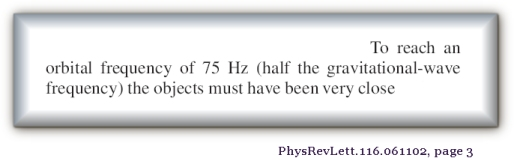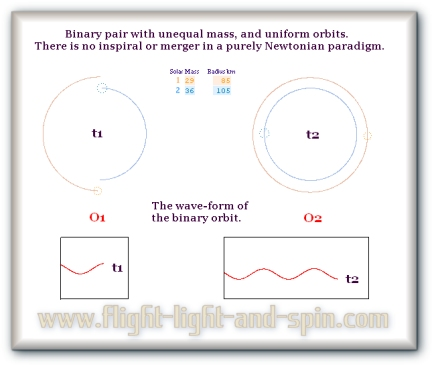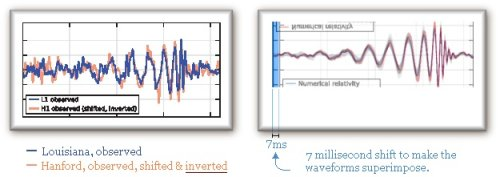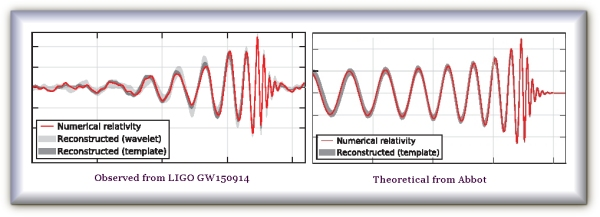The shape of the LIGO waveform

 1 2 3 4 5 6 7 8 9 10
 3 There is a fundamental problem that Abbot seems not to appreciate when it is said:This is a fatal error simply because gravitational-waves are not the orbital frequency. The orbital frequency has everything to do with the system being a binary. There would be no orbital frequency if it was a single object. Yet a single object is still supposed to emit gravitational-waves according to Hawking/Einstein where it replaces Newton’s instant gravity. It is appalling philosophy to confuse the orbital frequency of a binary system with a theory on the velocity of gravity. .
 I deliberately make a sharp semantic distinction between the term from Einstein of ‘gravitational-wave’, and the term ‘gravity wave-form of the orbit’. These terms are utterly different aspects of the theoretical physics and the math. To explain further why this distinction is so vital to this analysis, I first need to describe the ‘gravity wave-form of the orbit’ (not gravitational-waves) of a much simpler purely Newtonian example. The software (orbit-gravity-sim-11.exe or OGS11) demonstrates the relationship between the orbital frequency and the gravity wave-form in real-time. This can be observed in the evolutionary algorithm by selecting scenario . As the pair orbit one another anti-clockwise, their combined gravity pulls towards an observer at the bottom of the screen. In the graphs to follow, the wave-forms at the bottom thus move from left to right:Scenario  depicts a binary pair with unequal masses. The red graph-line is the sum total of the gravity of the binary pair pulling downwards towards the respective observers at O1, and O2. It can be seen that at the time t2 where the pair are horizontal, the pull is weaker than at the time t1 where the pair are vertical. At t2 the pull is less towards the observer O2 whereas at t1 the observer O1 experiences more gravity by comparison. So when the pair rotates, the graph oscillates with the pull of gravity in a regular wave-shape as viewed by the observers. Nonetheless, this is all purely to demonstrate that the gravity wave-form of the orbit for the binary pair is quite different to the theory of gravitational-waves. It would be very easy to mistakenly conflate the observed data of the ‘gravity wave-form of the orbit’ of the binary pair with the concept of ‘gravitational-wave’ because of semantic similarities in terminology. The wave-form in the observed LIGO data, still only represents the shape that is the result of the combined pull of gravity by the binary pair in orbit around each other. That shape is not the actual ‘gravitational-wave’ from General Relativity. The graphs previously are of course Newtonian, and are not directly related to General Relativity or gravitational-waves. .
 But the observed data of the gravity of the pair in GW150914 causes quite a different shape to this.So obviously there is something quite different happening with the LIGO observation. Abbot also demonstrates the merger process like this:The above diagram shows that the wave-form is a result of the orbits of the binary system. So we can clearly see that it appears as though a purely Newtonian dynamic is insufficient to describe the in-spiral and Ringdown. In the observed data we have an increase in amplitude before it flattens. But also note the discrepancy in the two official LIGO wave-forms from Abbott. Their second graph (theoretical) begins with amplitudes far higher than the first graph. The first graph (observed) is far flatter to begin with. This is quite a marked difference which shows that Abbot’s theoretical account for the observation does not quite fit the data….

 Next Page ->

^ Top of Page ^